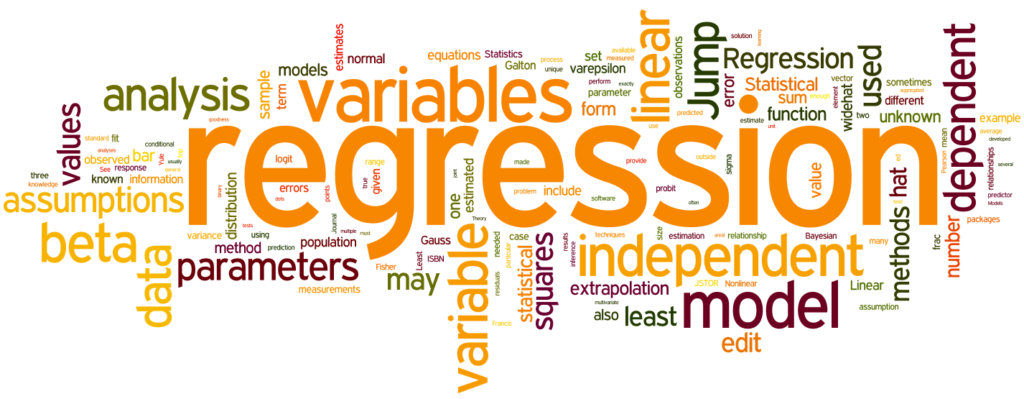# The most used regression algorithms that you should know about

0
804

The most used regression algorithms that you should know about: We implement a variety of algorithms in Machine Learning to allow machines to understand the data and generate predictions based on patterns or rules discovered in the data.As a result, regression is a machine learning approach in which the model predicts the outcome as a continuous numerical value. Regression algorithms are a subset of machine learning algorithms that belong to the Supervised Machine Learning algorithm. The algorithm generates a model based on the properties of training data and then utilizes the model to forecast the value of new data.

Regression models are now widely used in several fields, including economic forecasting, trend breakdown, marketing, time series projection, and medication response modeling.

Let us dive in to learn the most used regression algorithms in machine learning that you should know about

### Some of the most used regression algorithms

Simple Linear Regression model:

Users can analyze and study correlations between two continuous variables using the statistical method of simple linear regression. A linear model in which the input variables and a single output variable have a linear relationship is known as linear regression. The output can be calculated using a linear combination of the input variables. When there is just one input variable, the procedure is simple linear regression.

Also Read :   Indo Global College organized seminar & presentation on smart cities

Least Absolute Selection Shrinkage Operator Regression (LASSO):

Lasso regression is to identify the subset of predictors with the lowest prediction error for a quantitative response variable. The method works by setting a limit on the model parameters, causing some regression coefficients to drop to zero. After the shrinking method, variables with a regression coefficient of 0 are eliminated from the model. Variables with non-zero regression coefficients are tightly linked to the response variable.

Tree of Decisions

The decision tree models are used with any data that has both numerical and categorical properties. Decision trees will capture Non-linear interactions between characteristics and the goal variable. Because decision trees resemble human thought patterns, understanding the data becomes simple.

Logistic regression:

One of the company’s most often used regression procedures offers a significant benefit wherever the answer is binary. It’s used in several applications. One of the most appealing aspects of this well-known approach is that it allows for many dependent variables, which can be continuous or noncontinuous. Another key advantage of this supervised machine learning method is that it generates a quantitative value that can be used to analyze the degree of correlation between the variables.

Support Vector Machines

SVM has high regularization and is utilized for classification and regression problems is another powerful supervised machine learning approach. The system’s capacity is governed by parameters that are independent of the dimensionality of the feature space. As it functions natively on numeric attributes, the SVM approach uses a z-score normalization. Support Vector Machines techniques employ the margin of the tolerance loss function’s to tackle regression difficulties.

Also Read :   Amway India celebrates International White Cane Day with National Association for the Blind

Multivariate Regression algorithm

When a regression model has more than one predictor variable, this approach is used, and the model is called a multivariate multiple regression. This regression machine learning algorithm is one of the simplest supervised machine learning algorithms for predicting the response variable given a set of explanatory factors. Matrix operations can be leveraged to accomplish this regression strategy, and the “NumPy” package in Python provides explanations and methods for matrix objects.

Multiple Regression Algorithm:

This regression technique is used in various industries for pricing policy, real estate pricing, and marketing departments to determine the effectiveness of programs. Multiple regression is a larger class of regressions that includes linear and nonlinear regressions with multiple explanatory variables compared to linear regression algorithms.

Conclusion

Regression models are leveraged in almost all the sectors of the marketplace. These are the most used regression algorithms. To explore more about regression algorithms in machine learning, Check out the jigsaw machine learning course to increase your machine learning knowledge.